# VS2010 CUDA8.0 工程配置

1.打开vs2010,创建win32控制台项目,命名为cuda_test,点确定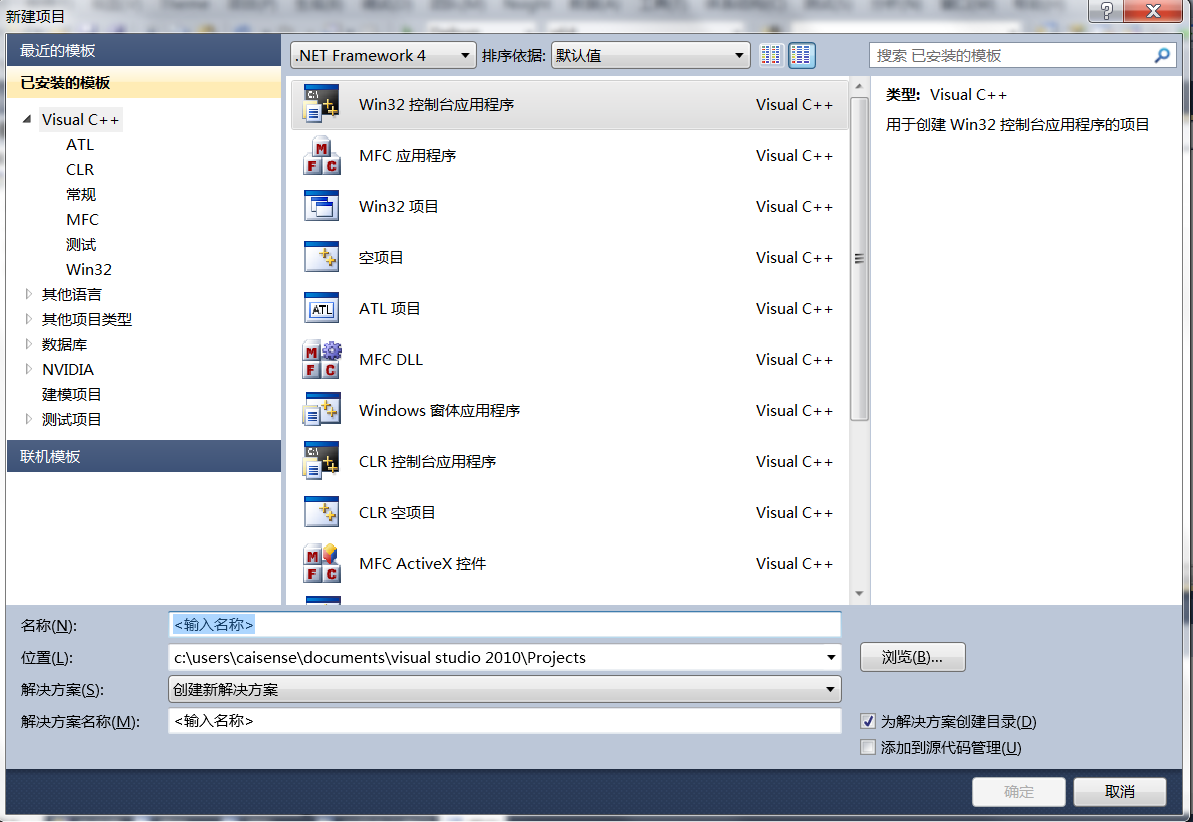2.勾选空项目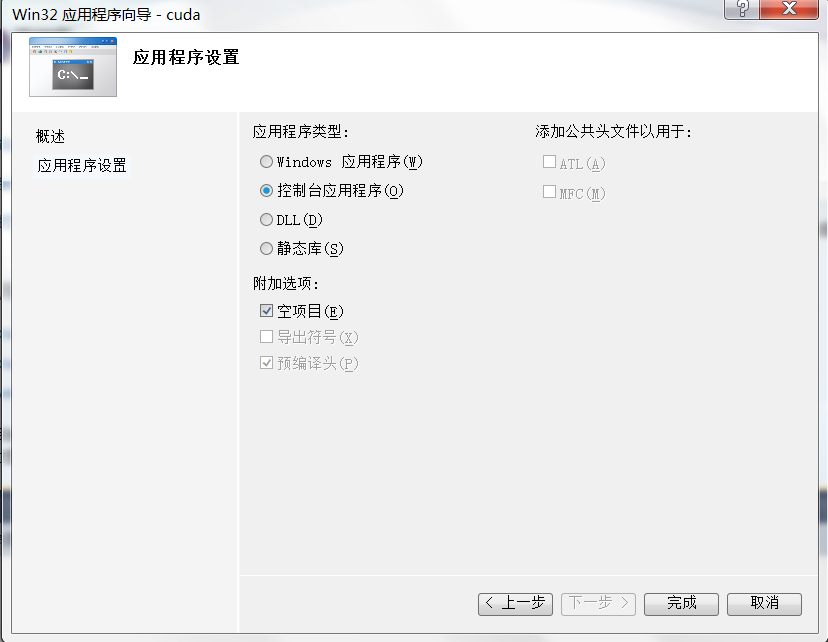3.右键”源文件”目录,选择 “添加–新建项”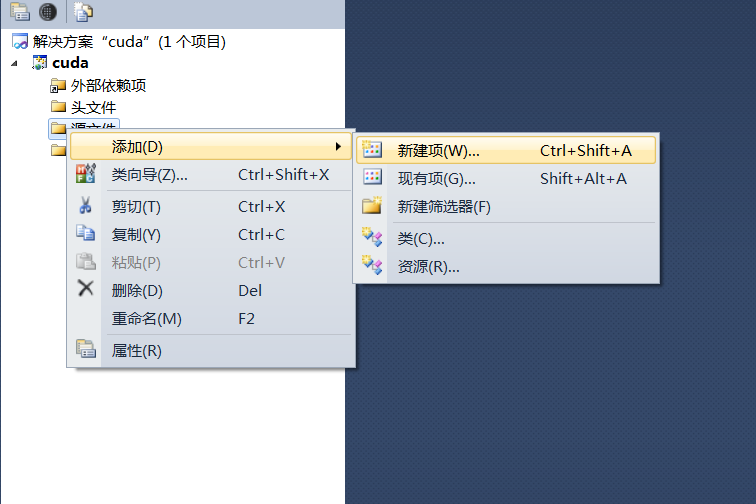4.左侧选择NVIDIA CUDA8.0,在中间图标处选择CUDA/C file,命名为test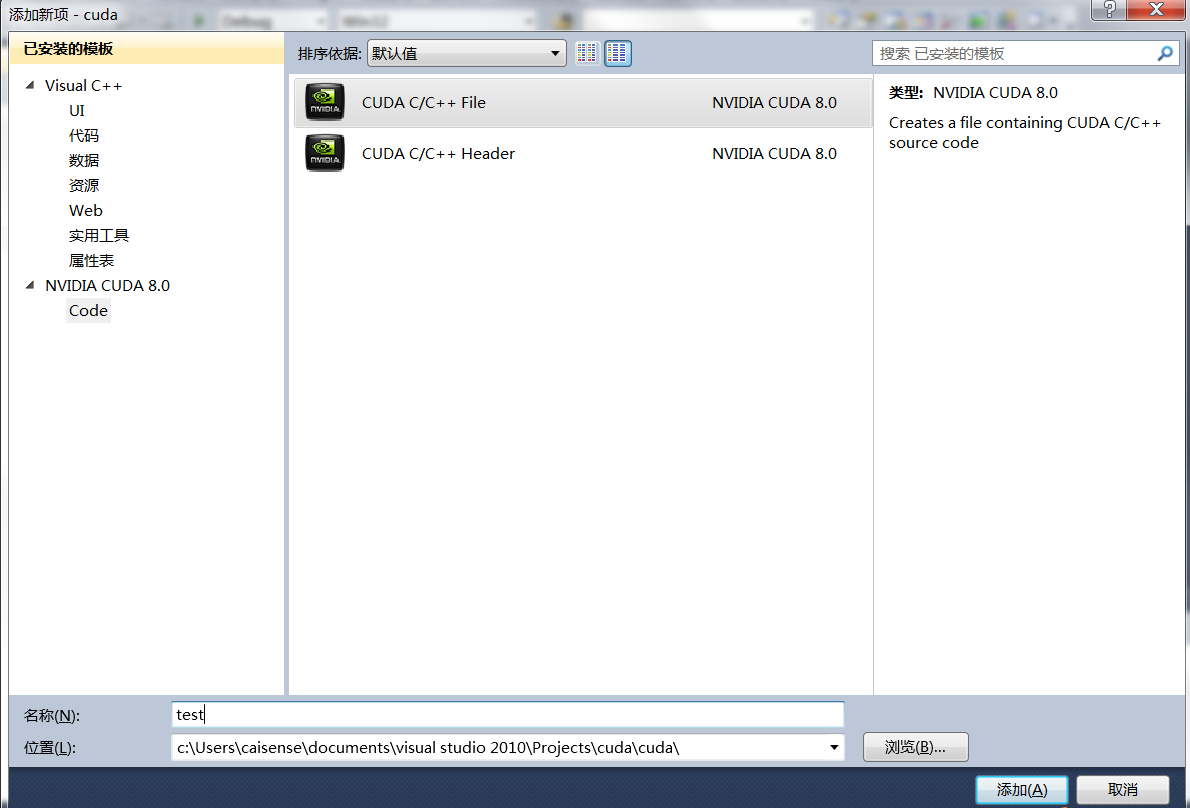5.右键cuda项目–生成自定义

6.勾选CUDA8.0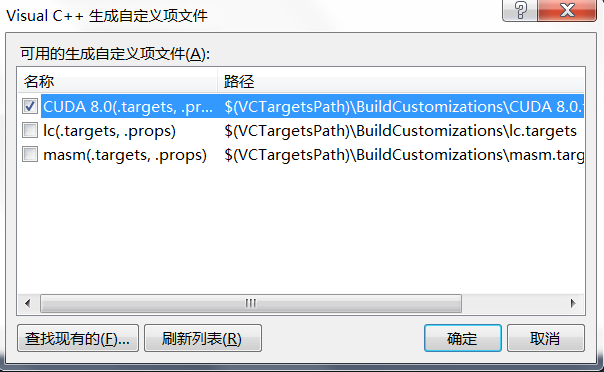7.右键刚才创建的test.cu文件.左侧选”配置属性–常规”,在右边选择项类型,下拉菜单中选CUDA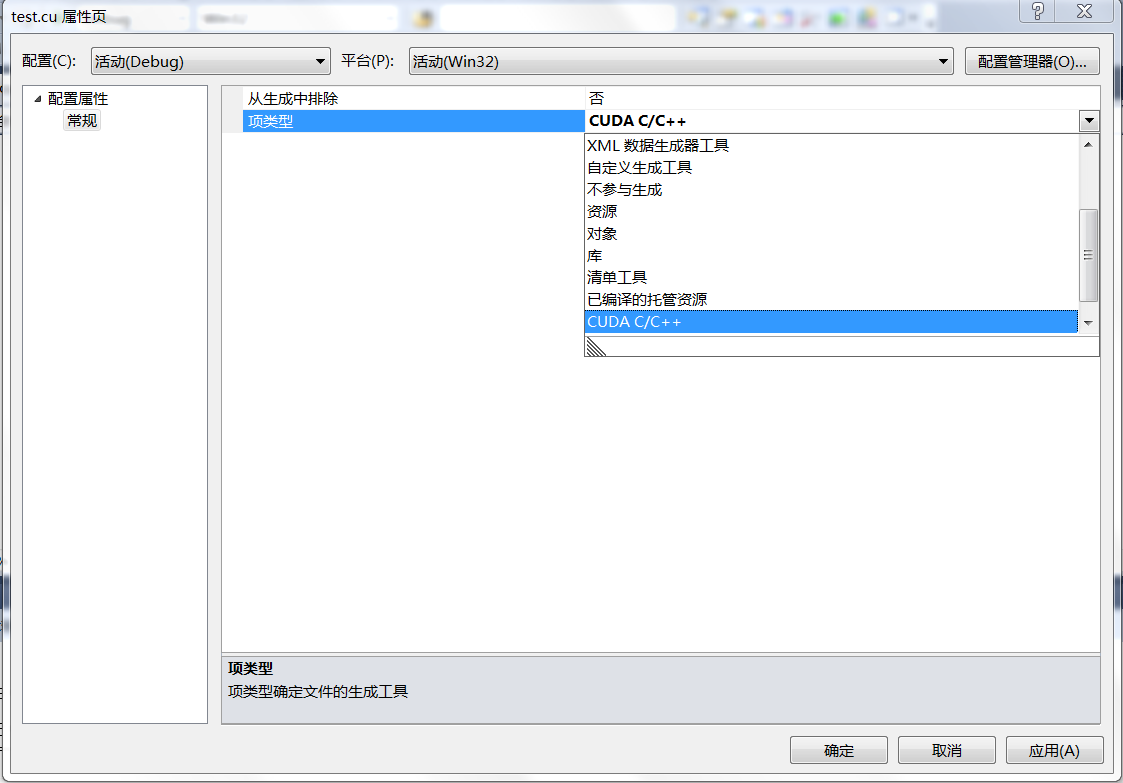8.在test.cu文件中添加cuda代码(见文末)

9.右键cuda项目——属性——配置属性——CUDA C/C++——常规——附加包含目录，增加一项:
C:\ProgramData\NVIDIA Corporation\CUDA Samples\v8.0\common\inc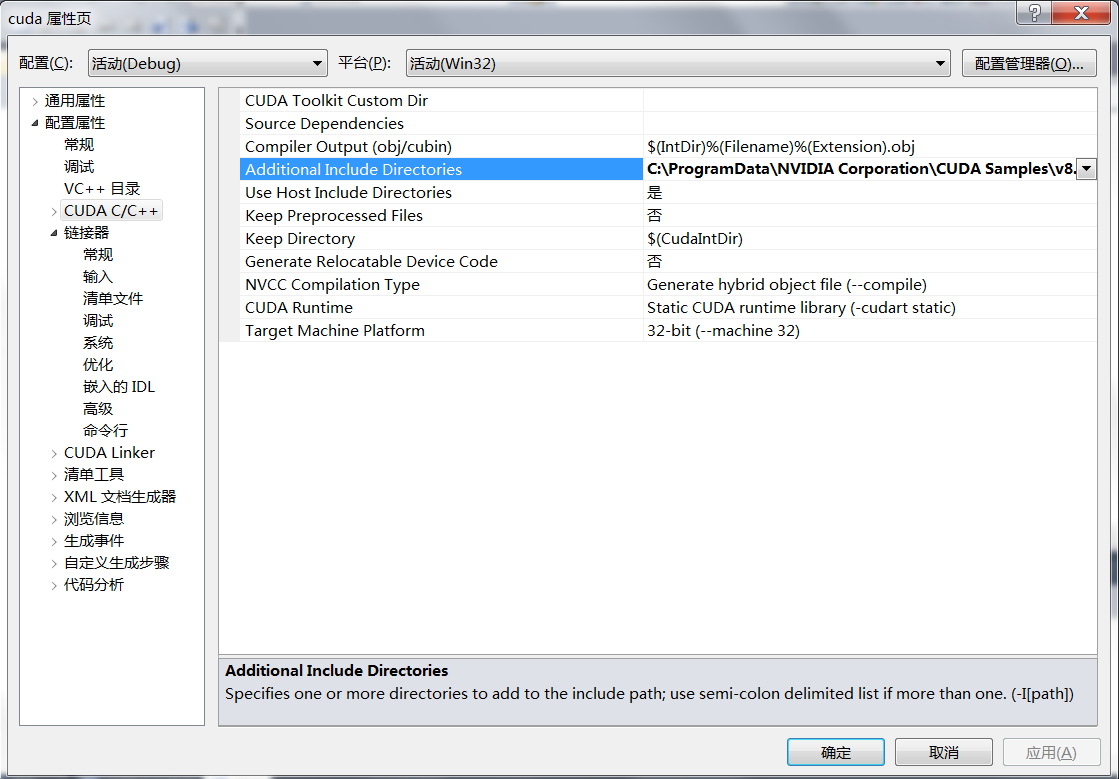<helper_string.h>
<helper_cuda.h>
<helper_functions.h>

C:\ProgramData\NVIDIACorporation\CUDA Samples\v8.0\common\inc

10.在链接器–输入–附加依赖项,点击下拉编辑,添加一条cudart.lib,否则会报错无法生成项目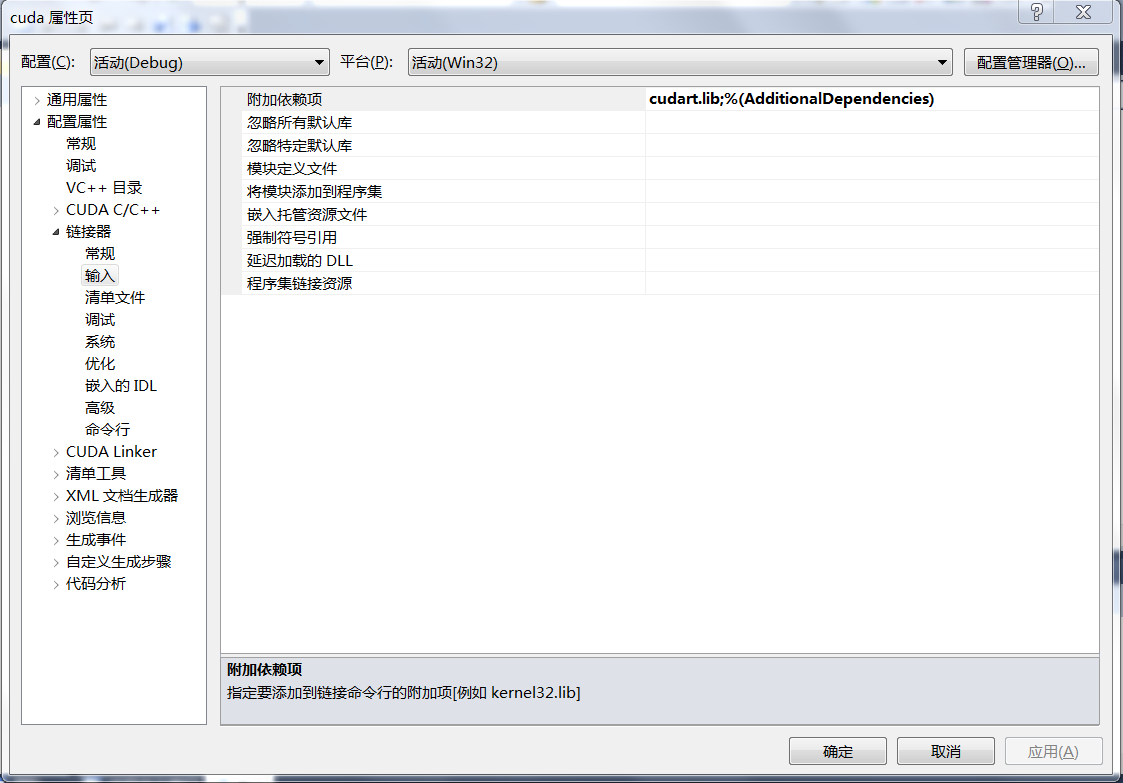11.开始运行,如图所示则创建成功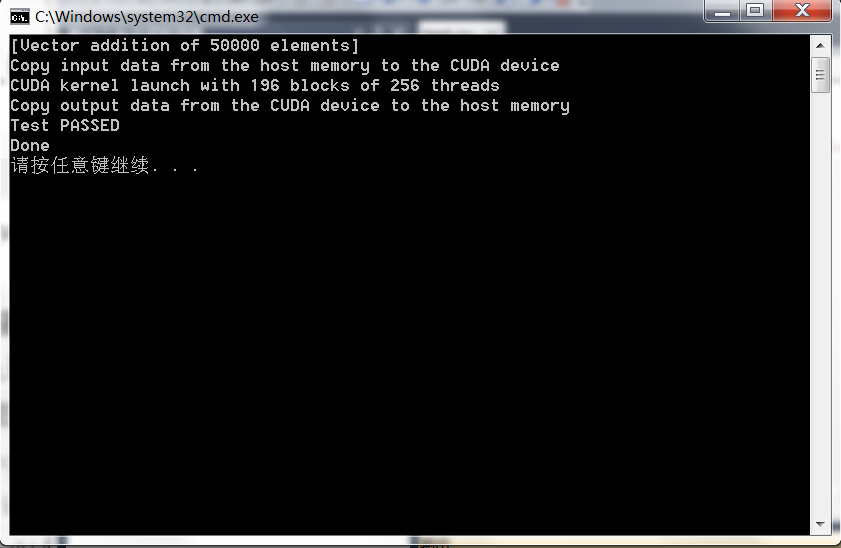(引用自cuda源码示例的vectorAdd)

/**
* Copyright 1993-2015 NVIDIA Corporation.  All rights reserved.
*
* Please refer to the NVIDIA end user license agreement (EULA) associated
* with this source code for terms and conditions that govern your use of
* this software. Any use, reproduction, disclosure, or distribution of
* this software and related documentation outside the terms of the EULA
* is strictly prohibited.
*
*/

/**
* Vector addition: C = A + B.
*
* This sample is a very basic sample that implements element by element
* vector addition. It is the same as the sample illustrating Chapter 2
* of the programming guide with some additions like error checking.
*/

#include <stdio.h>

// For the CUDA runtime routines (prefixed with "cuda_")
#include <cuda_runtime.h>

#include <helper_cuda.h>
/**
* CUDA Kernel Device code
*
* Computes the vector addition of A and B into C. The 3 vectors have the same
* number of elements numElements.
*/
__global__ void
vectorAdd(const float *A, const float *B, float *C, int numElements)
{
int i = blockDim.x * blockIdx.x + threadIdx.x;

if (i < numElements)
{
C[i] = A[i] + B[i];
}
}

/**
* Host main routine
*/
int
main(void)
{
// Error code to check return values for CUDA calls
cudaError_t err = cudaSuccess;

// Print the vector length to be used, and compute its size
int numElements = 50000;
size_t size = numElements * sizeof(float);
printf("[Vector addition of %d elements]\n", numElements);

// Allocate the host input vector A
float *h_A = (float *)malloc(size);

// Allocate the host input vector B
float *h_B = (float *)malloc(size);

// Allocate the host output vector C
float *h_C = (float *)malloc(size);

// Verify that allocations succeeded
if (h_A == NULL || h_B == NULL || h_C == NULL)
{
fprintf(stderr, "Failed to allocate host vectors!\n");
exit(EXIT_FAILURE);
}

// Initialize the host input vectors
for (int i = 0; i < numElements; ++i)
{
h_A[i] = rand()/(float)RAND_MAX;
h_B[i] = rand()/(float)RAND_MAX;
}

// Allocate the device input vector A
float *d_A = NULL;
err = cudaMalloc((void **)&d_A, size);

if (err != cudaSuccess)
{
fprintf(stderr, "Failed to allocate device vector A (error code %s)!\n", cudaGetErrorString(err));
exit(EXIT_FAILURE);
}

// Allocate the device input vector B
float *d_B = NULL;
err = cudaMalloc((void **)&d_B, size);

if (err != cudaSuccess)
{
fprintf(stderr, "Failed to allocate device vector B (error code %s)!\n", cudaGetErrorString(err));
exit(EXIT_FAILURE);
}

// Allocate the device output vector C
float *d_C = NULL;
err = cudaMalloc((void **)&d_C, size);

if (err != cudaSuccess)
{
fprintf(stderr, "Failed to allocate device vector C (error code %s)!\n", cudaGetErrorString(err));
exit(EXIT_FAILURE);
}

// Copy the host input vectors A and B in host memory to the device input vectors in
// device memory
printf("Copy input data from the host memory to the CUDA device\n");
err = cudaMemcpy(d_A, h_A, size, cudaMemcpyHostToDevice);

if (err != cudaSuccess)
{
fprintf(stderr, "Failed to copy vector A from host to device (error code %s)!\n", cudaGetErrorString(err));
exit(EXIT_FAILURE);
}

err = cudaMemcpy(d_B, h_B, size, cudaMemcpyHostToDevice);

if (err != cudaSuccess)
{
fprintf(stderr, "Failed to copy vector B from host to device (error code %s)!\n", cudaGetErrorString(err));
exit(EXIT_FAILURE);
}

// Launch the Vector Add CUDA Kernel
int threadsPerBlock = 256;
int blocksPerGrid =(numElements + threadsPerBlock - 1) / threadsPerBlock;
printf("CUDA kernel launch with %d blocks of %d threads\n", blocksPerGrid, threadsPerBlock);
vectorAdd<<<blocksPerGrid, threadsPerBlock>>>(d_A, d_B, d_C, numElements);
err = cudaGetLastError();

if (err != cudaSuccess)
{
fprintf(stderr, "Failed to launch vectorAdd kernel (error code %s)!\n", cudaGetErrorString(err));
exit(EXIT_FAILURE);
}

// Copy the device result vector in device memory to the host result vector
// in host memory.
printf("Copy output data from the CUDA device to the host memory\n");
err = cudaMemcpy(h_C, d_C, size, cudaMemcpyDeviceToHost);

if (err != cudaSuccess)
{
fprintf(stderr, "Failed to copy vector C from device to host (error code %s)!\n", cudaGetErrorString(err));
exit(EXIT_FAILURE);
}

// Verify that the result vector is correct
for (int i = 0; i < numElements; ++i)
{
if (fabs(h_A[i] + h_B[i] - h_C[i]) > 1e-5)
{
fprintf(stderr, "Result verification failed at element %d!\n", i);
exit(EXIT_FAILURE);
}
}

printf("Test PASSED\n");

// Free device global memory
err = cudaFree(d_A);

if (err != cudaSuccess)
{
fprintf(stderr, "Failed to free device vector A (error code %s)!\n", cudaGetErrorString(err));
exit(EXIT_FAILURE);
}

err = cudaFree(d_B);

if (err != cudaSuccess)
{
fprintf(stderr, "Failed to free device vector B (error code %s)!\n", cudaGetErrorString(err));
exit(EXIT_FAILURE);
}

err = cudaFree(d_C);

if (err != cudaSuccess)
{
fprintf(stderr, "Failed to free device vector C (error code %s)!\n", cudaGetErrorString(err));
exit(EXIT_FAILURE);
}

// Free host memory
free(h_A);
free(h_B);
free(h_C);

printf("Done\n");
return 0;
}